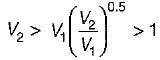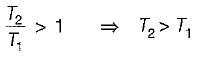Courses

# Test: Properties of Gases - 3

## 15 Questions MCQ Test Topicwise Question Bank for Mechanical Engineering | Test: Properties of Gases - 3

Description
This mock test of Test: Properties of Gases - 3 for Mechanical Engineering helps you for every Mechanical Engineering entrance exam. This contains 15 Multiple Choice Questions for Mechanical Engineering Test: Properties of Gases - 3 (mcq) to study with solutions a complete question bank. The solved questions answers in this Test: Properties of Gases - 3 quiz give you a good mix of easy questions and tough questions. Mechanical Engineering students definitely take this Test: Properties of Gases - 3 exercise for a better result in the exam. You can find other Test: Properties of Gases - 3 extra questions, long questions & short questions for Mechanical Engineering on EduRev as well by searching above.
QUESTION: 1

### The characteristic equation of gases PV = mRT holds good for

Solution:

Pv= mRT is known as ideal gas equation.

QUESTION: 2

Solution:
QUESTION: 3

### Variation of pressure and volume at constant temperature are correlated through

Solution:

As per Boyle’s law
At constant temperature
PV = Constant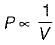QUESTION: 4

Polytropic index n is given by

Solution:
QUESTION: 5

The work given out during expansion process in a closed system will increase when the value of n (the index of compression)

Solution:
QUESTION: 6

The work required for compression in a closed system increases the value of n (the index of compression)

Solution:
QUESTION: 7

In thermodynamics analysis, a pure substance is that which

Solution:
QUESTION: 8

Which one of the following PV - T diagram correctly represents that of an ideal gas?

Solution:
QUESTION: 9

If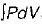and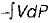for a thermodynamic system of an ideal gas on evaluation give the same quantify during a process, then the process under gone by the system is

Solution:

For isothermal process,
PV = Constant
PdV + VdP = 0
∵ PdV = - VdP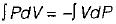QUESTION: 10

A vessel of volume 1m3 contains oxygen (molecular weight = 32) at P = 1 bar and T = 47°C. The mass of oxygen in the vessel is (take universal gas constant as 8314 J/mol-K)

Solution: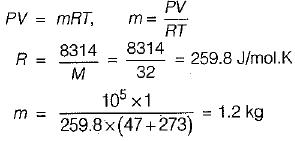QUESTION: 11

Nitrogen at an initial state of 10 bar, 1 m3, and 300 K is expanded isothermally to a final volume of 2m3. The p-v-T relation is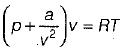, where a > 0. The final pressure.

Solution:

For isothermal process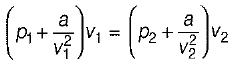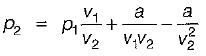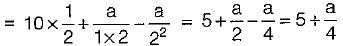Hence final pressure will be slightly more than 5 bar as a is + ve.

QUESTION: 12

Which combination of the following statements is correct?
P : A gas cools upon expansion only when its Joule-Thomson coefficient is positive in the temperature range of expansion.
Q : For a system undergoing a process, its entropy remains constant only when the process is reversible.
R : The work done by a closed system in an adiabatic is a point function S : A liquid expands upon freezing when the slope of its fusion curve on pressure-temperature diagram is negative.

Solution:
QUESTION: 13

Van der Waal’s equation of state is given by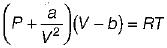The value of R in terms of Pc, Vc & Tc is

Solution:
QUESTION: 14

The equation of state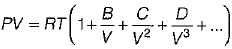is known as

Solution:

Virial expansion is given as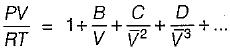QUESTION: 15

An ideal gas undergoes expansion according to the process PV0.5 = constant. The temperature of'the gas during expansion process

Solution: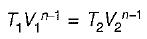∴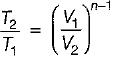for expansion process, V> V1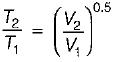as